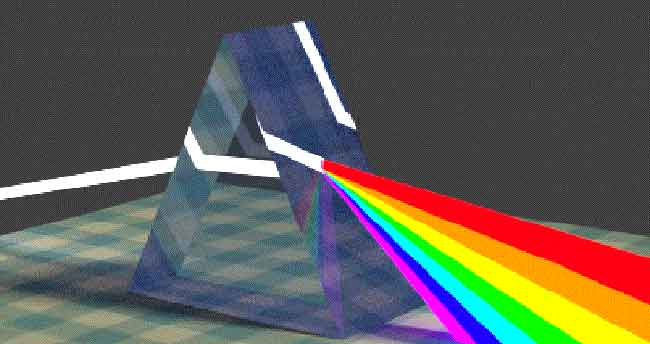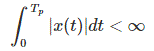# Fourier Series and Fourier Transform

Sometimes all the information in time domain is not sufficient. This makes us to move to frequency domain of the signal for extracting more information about the signal. This movement from one domain to other domain is known as transformation. For changing the domain of signal from time to frequency we have many tools. Fourier Series and Fourier Transform are two of the tools in which we decompose the signal into harmonically related sinusoids. With such decomposition, a signal is said to be represented in frequency domain.
Most of the practical signals can be decomposed into sinusoids. Such a decomposition of periodic signals is called a Fourier series.

## Frequency Analysis

Just like a white light can be decomposed into seven colors, a periodic signal can also be decomposed into a liner weighted sum of harmonically related frequencies. This linear weighted sum of harmonically related sinusoids or complex exponentials is known as Fourier Series or Transform. In general, decomposition of any signal into its frequency related components is called frequency analysis. Like analysis of a light into colors is actually a form of frequency analysis, hence the Fourier series and Fourier transform are also tools of frequency analysis.

This can be clearer from the following.
Suppose if we pass a light through a prism, it gets split into seven colors VIBGYOR. Each color has a particular frequency or a range of frequencies. In the same way, if we pass a periodic signal through a Fourier tool, which plays the role of prism, the signal is decomposed into a Fourier series.### Signals and Vectors Analogy

An N dimension vector needs N dimensions for its representation. Like an ant moving on a table needs two dimensions for the representation of its position on the table i.e. x and y. Also we are familiar with i, j, k coordinate system for a vector representation in three dimensions. This unit vector i, j and k are orthogonal to each other. In the same way if we treat a signal as a multidimensional vector we need many more dimensions which are orthogonal to each other. It was the genius of J. B. J. Fourier who invented multi-dimensions, which are orthogonal to each other. These are sinusoids with harmonically related sinusoids or complex exponential. Consider the dimensions (also called bases)
sinω0t sin2ω0t sin3ω0t sin4ω0t ……..sinnω0t
cosω0t cos2ω0t cos3ω0t cos4ω0t……..cosnω0t
Thus, all sinnω0t are orthogonal with Sinmω0t (n≠m) and we, therefore can use sinω0t, sin2ω0t… ∞ as the primary dimensions (also called bases) to express a periodic signal. Similarly, we can also use cosω0t, cos2ω0t, cos3ω0t… ∞ as the additional dimensions when sinω0t dimensions cannot be used. We will see for even signals only cosine terms will be suitable and for odd signal only sine terms will be suitable. For a periodic signal neither an odd nor even, we use both sine and cosine terms.

NOTE
Only periodic signals can be represented as Fourier series provided the signal follows the Dirichlet’s conditions. For non-periodic signals, we have Fourier transform tool which transform the signal from time domain to frequency domain.
Resolution of signal into its harmonically related frequencies is known as Fourier Analysis while the inverse i.e. recombination, is known as Fourier Sysnthesis.

### Dirichlet’s Conditions

x (t) is absolutely integrable over any period, that is,x (t) has a finite number of maxima and minima within any finite interval of t.
x (t) has a finite number of discontinuities within any finite interval of t, and each of these discontinuities are finite.
Note that the Dirichlet’s conditions are sufficient but not necessary conditions for the Fourier series representation.

Want To Learn Faster? 🎓
Get electrical articles delivered to your inbox every week.
No credit card required—it’s 100% free.